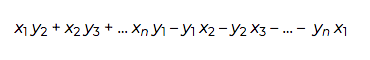{{announcement.body}}
{{announcement.title}}

# Surveyors Formula for Area of a Polygon

DZone 's Guide to

# Surveyors Formula for Area of a Polygon

### In this post, a math whiz and Python developer demonstrates the scientific power of Python and the NumPy library by using them to calculate the area of a polygon.

· Big Data Zone ·
Free Resource

Comment (0)

Save
{{ articles.views | formatCount}} Views

If you know the vertices of a polygon, how do you compute its area? This seems like this could be complicated, with special cases for whether the polygon is convex or maybe other considerations. But as long as the polygon is "simple," i.e. the sides meet at vertices but otherwise do not intersect each other, then there is a general formula for the area.

## The FormulaBut what does that mean? The notation is meant to be suggestive of a determinant. It's not literally a determinant because the matrix isn't square. But you evaluate it in a way analogous to 2 by 2 determinants: add the terms going down and to the right, and subtract the terms going up and to the right. That is:This formula is sometimes called the shoelace formula because the pattern of multiplications resembles lacing a shoe. It's also called the surveyor's formula because it's obviously useful in surveying.

## Numerical Implementation

As someone pointed out in the comments, in practice you might want to subtract the minimum x value from all the x coordinates and the minimum y value from all the y coordinates before using the formula. Why's that?

If you add a constant amount to each vertex, you move your polygon but you don't change the area. So, in theory, it makes no difference whether you translate the polygon before computing its area. But in floating point, it can make a difference.

The cardinal rule of floating point arithmetic is to avoid subtracting nearly equal numbers. If you subtract two numbers that agree to k significant figures, you can lose up to k figures of precision. We'll illustrate this by taking a right triangle with base 2 and height π. The area should remain π as we translate the triangle away from the origin, we lose precision the further out we translate it.

Here's a Python implementation of the shoelace formula.

``````    def area(x, y):
n = len(x)
s = 0.0
for i in range(-1, n-1):
s += x[i]*y[i+1] - y[i]*x[i+1]
return 0.5*s``````

If you're not familiar with Python, a couple things require explanation. First, `range(n-1)` is a list of integers starting at 0 but less than n-1. Second, the -1 index returns the last element of the array.

Now, watch how the precision of the area degrades as we shift the triangle by powers of 10.

``````    import numpy as np

x = np.array([0.0, 0.0, 2.0])
y = np.array([np.pi, 0.0, 0.0])

for n in range(0, 10):
t = 10**n
print( area(x+t, y+t) )``````

This produces:

``````    3.141592653589793
3.1415926535897825
3.1415926535901235
3.1415926535846666
3.141592651605606
3.1415929794311523
3.1416015625
3.140625
3.0
0.0``````

Shifting by small amounts doesn't make a noticeable difference, but we lose between one and two significant figures each time we increase t by a multiple of 10. We only have between 15 and 16 significant figures to start within a standard floating point number, and so eventually we completely run out of significant figures.

When implementing the shoelace formula, we want to do the opposite of this example: instead of shifting coordinates so that they're similar in size, we want to shift them toward the origin so that they're not similar in size.

Topics:
big data ,numpy ,python ,tutorial

Comment (0)

Save
{{ articles.views | formatCount}} Views

Published at DZone with permission of John Cook , DZone MVB. See the original article here.

Opinions expressed by DZone contributors are their own.